# Connected component of the identity

(diff) ← Older revision | Latest revision (diff) | Newer revision → (diff)
identity component, of a groupThe largest connected subset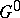of the topological (or algebraic) groupthat contains the identity element of this group. The componentis a closed normal subgroup of; the cosets with respect tocoincide with the connected components of. The quotient group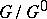is totally disconnected and Hausdorff, andis the smallest among the normal subgroups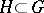such that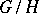is totally disconnected. Ifis locally connected (for example, ifis a Lie group), thenis open inandis discrete.
In an arbitrary algebraic groupthe identity componentis also open and has finite index; also,is the minimal closed subgroup of finite index in. The connected components of an algebraic groupcoincide with the irreducible components. For every polynomial homomorphismof algebraic groups one has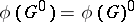. Ifis defined over a field, thenis defined over this field.
Ifis an algebraic group over the field, then its identity componentcoincides with the identity component ofconsidered as a complex Lie group. Ifis defined over, then the groupof real points inis not necessarily connected in the topology of the Lie group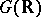, but the number of its connected components is finite. For example, the group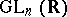splits into two components, althoughis connected. The pseudo-orthogonal unimodular group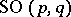, which can be regarded as the group of real points of the connected complex algebraic group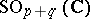, is connected for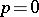or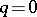, and splits into two components for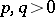. However, if the Lie groupis compact, thenis connected.